Next: Generalized Forces Up: Lagrangian Dynamics Previous: Introduction

Generalized Coordinates

Let the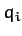, for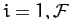, be a set of coordinates which uniquely specifies the instantaneous configuration of some dynamical system. Here, it is assumed that each of thecan vary independently. Themight be Cartesian coordinates, or polar coordinates, or angles, or some mixture of all three types of coordinate, and are, therefore, termed generalized coordinates. A dynamical system whose instantaneous configuration is fully specified by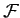independent generalized coordinates is said to havedegrees of freedom. For instance, the instantaneous position of a particle moving freely in three dimensions is completely specified by its three Cartesian coordinates,,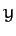, and. Moreover, these coordinates are clearly independent of one another. Hence, a dynamical system consisting of a single particle moving freely in three dimensions has three degrees of freedom. If there are two freely moving particles then the system has six degrees of freedom, and so on.

Suppose that we have a dynamical system consisting of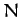particles moving freely in three dimensions. This is an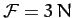degree of freedom system whose instantaneous configuration can be specified byCartesian coordinates. Let us denote these coordinates the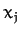, for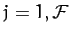. Thus,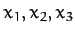are the Cartesian coordinates of the first particle,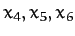the Cartesian coordinates of the second particle, etc. Suppose that the instantaneous configuration of the system can also be specified bygeneralized coordinates, which we shall denote the, for. Thus, themight be the spherical coordinates of the particles. In general, we expect theto be functions of the. In other words,(592)

for. Here, for the sake of generality, we have included the possibility that the functional relationship between theand themight depend on the time,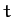, explicitly. This would be the case if the dynamical system were subject to time varying constraints. For instance, a system consisting of a particle constrained to move on a surface which is itself moving. Finally, by the chain rule, the variation of thedue to a variation of the(at constant) is given by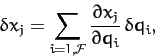(593)

for.Next: Generalized Forces Up: Lagrangian Dynamics Previous: Introduction
Richard Fitzpatrick 2011-03-31